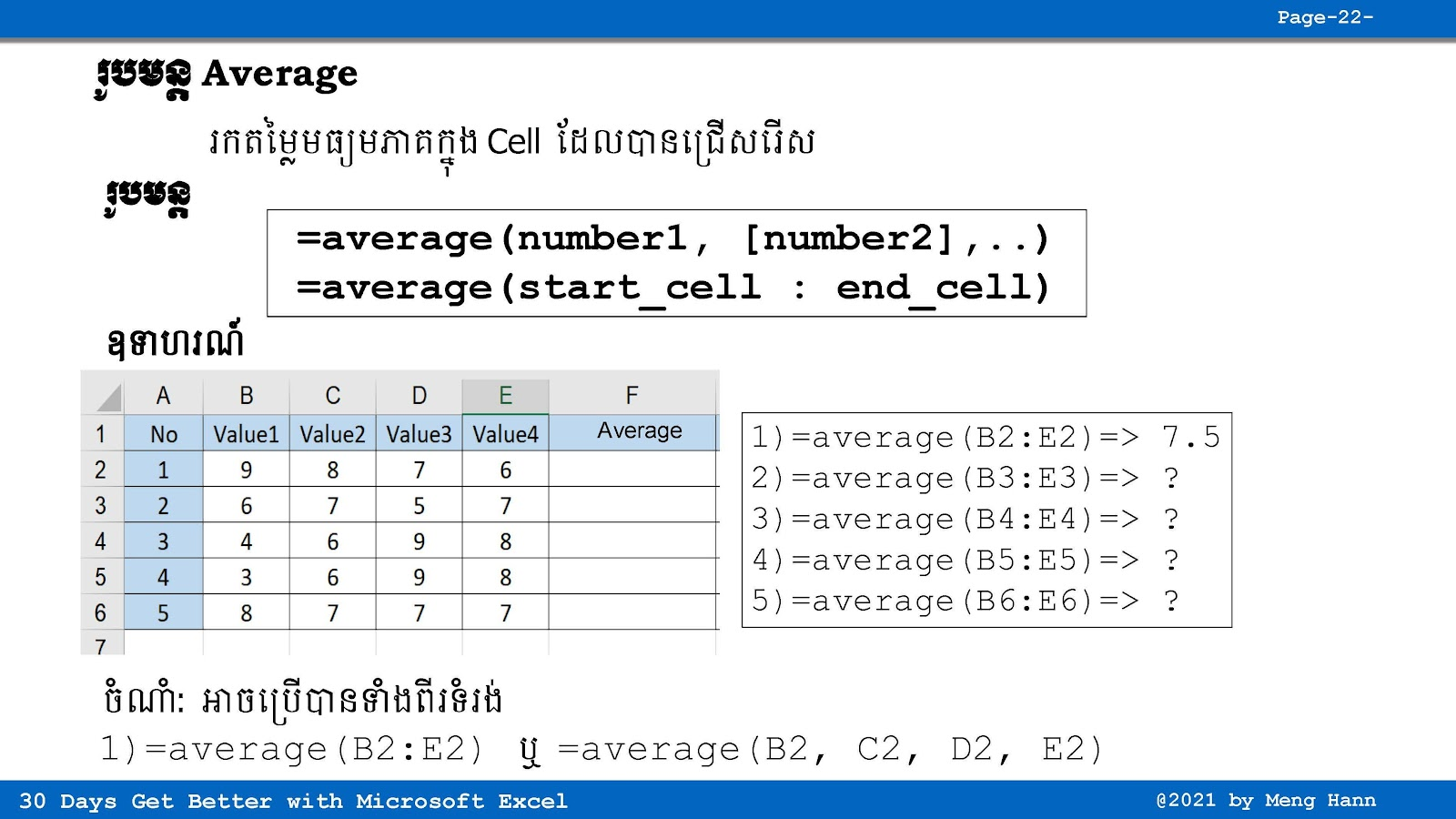# 15. How to use Average in Excel speak Khmer

15. How to use Average in Excel speak Khmer
(រូបមន្ត Average) this Excel tutorial shows step by step how to use average formula to calucate average value in Excel. Average Formula: find average value of selected cells
=Average(Number1,[Number2],...)
=Average(start_cell:end_cell)
◾ Number1: Required, value to calculate, (number1,...,number255)
◾ start_cell: Required, start value to calculate.
◾ end_cell: Required, end value to calculate.